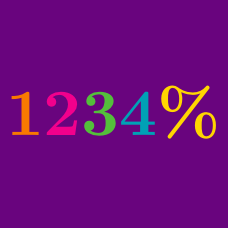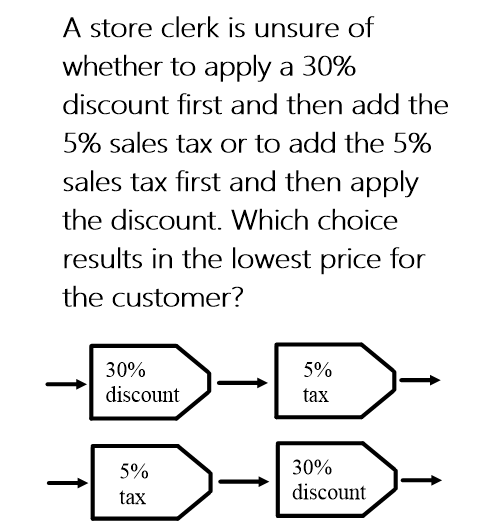Basic Mathematics

# Percentages

## Intro

Percentage, written as %, started as simply as “per cento” or “parts per one hundred”. This simple invention provides a simple and intuitive way to think of fractions, but still traps the unwary.

Expect to see and learn how to solve questions like this one:What happens when you multiply percentages? Or divide them? Or apply a percentage for multiple times? These questions and more are addressed here.

×